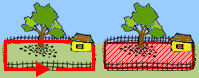# Challenge Exercises: Perimeter & AreaDirections: Read each question below. Click once in an ANSWER BOX and type in your answer; then click ENTER. Your answers should be given as whole numbers greater than zero. After you click ENTER, a message will appear in the RESULTS BOX to indicate whether your answer is correct or incorrect. To start over, click CLEAR.

 1.A piece of paper has a length of 16 inches and an area of 48 square inches. Find the width.  ANSWER BOX:=  in  RESULTS BOX:
 2.The perimeter of a square-shaped rug is 36 meters. Find the area.  ANSWER BOX:=  m2  RESULTS BOX:
 3.The area of a triangular-shaped garden is 72 square feet and the height is 24 feet. Find the base.  ANSWER BOX:=  ft  RESULTS BOX:
 4.The height of a parallelogram is 6 more units than the base. What is the area of the parallelogram if the base is 15 centimeters?  ANSWER BOX:=  cm2  RESULTS BOX:
 5.A dining room table has 8 sides of equal length. If one side measures 15 inches, what is the perimeter of the table?  ANSWER BOX:=  in  RESULTS BOX:
 6.A man has 30 feet of fence for a rectangular garden. What is the area of the largest garden he can build with whole-number dimensions?  ANSWER BOX:=  ft2  RESULTS BOX:
 7.A cardboard cut-out has 5 sides of equal length. If the perimeter is 65 centimeters, what is the length of one side?  ANSWER BOX:=  cm  RESULTS BOX:
 8.A chess board has an area of 49 square inches. Find the perimeter.  ANSWER BOX:=  in  RESULTS BOX:
 9.Room carpet costs \$25 per square yard. If the area of a bedroom is 20 square yards, how much will the room carpet cost? (No decimal point)  ANSWER BOX: \$   RESULTS BOX:
 10.A mirrored plate has 6 sides of equal length. If the perimeter is 72 centimeters, what is the length of one side?  ANSWER BOX:=  cm  RESULTS BOX: